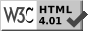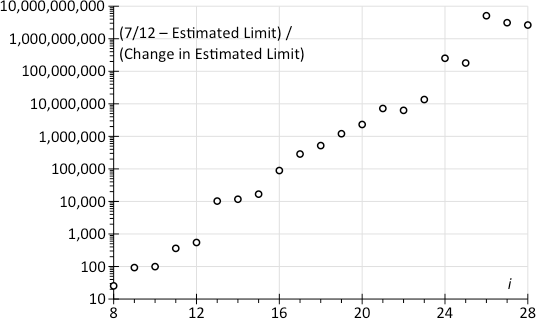# The Chow & Robbins Problem: Stop at h=5 t=3

Julian D. A. WisemanPublication history: only here. Usual disclaimer and copyright terms apply.

## • Introduction

1. The Chow & Robbins problem asks for the fair value of a game, in which tosses a coin, at least once, until choosing to stop, when one scores the proportion of tosses that are heads. An essay of late 2005, The expected value of sn/n ≈ 0.79295350640…, improved the best known estimate of the value of the game to ≈11½ decimal places. But it did not address any outstanding questions about possible stopping states.

Luis A. Medina & Doron Zeilberger, in ‘An Experimental Mathematics Perspective on the Old, and still Open, Question of When To Stop?’, arXiv:0907.0032v1, ask:

If currently you have five heads and three tails, should you stop?

If you stop, you can definitely collect 5/8 = 0.625, whereas if you keep going, your expected gain is > 0.6235, but no one currently knows to prove that it would not eventually exceeds 5/8 (even though this seems very unlikely, judging by numerical heuristics).

The author provides strong evidence that at h=5 t=4 the value of the game is ≈ 0.580572488…, and hence the value from continuing from h=5 t=3 is the average of this and 6÷9, that average being ≈ 0.623619577, which is significantly less than 0.625. Further, it seems likely that the error in the estimate of EV(h=5, t=4) is less than 10−10, so h=5 t=3 really is a stopping state. These further heuristics are consistent with the existing “numerical heuristics” cited by Medina & Zeilberger.

2. The previous code was constrained by memory. Code has been rewritten to remove this constraint. Extending the calculation by a factor of 4 has not improved the accuracy to which the value of the game is known. Current constraints definitely include CPU time, and also perhaps the accuracy of the hardware’s floating-point arithmetic.

## • 1. Whether to stop at h=5 t=3

With five heads and three tails, should one stop (scoring ⅝ = 0.625), or should one continue? Rephrased, is 0.625 ≥ ½(EV(6,3) + EV(5,4))? For n as large as has been tested, h=5 t=3 is a stopping state, so it is safe to assume that, with an extra head, so at h=6 t=3, one should definitely stop, there scoring 6/(6+3) = ⅔ ≈ 0.666666. So the question is equivalent to asking whether EV(5,4) ≤ 712 ≈ 0.583333.

The same technique previously used for the value of the whole game can be used to value the game at h=5 t=4. Results are output in the table that follows (new code available from jdawiseman.com/papers/easymath/chow_robbins.c; calculations run on a 1.83 GHz Intel Core 2 Duo processor under Mac OSX 10.6.4 using Xcode 3.2.3).

in = 2iEVn(h=5, t=4)Diff EVRatio
Diff EV
Estimated limit =
EV + Diff /
(1Ratio − 1)
Diff
Estimated limit
Diff
Estimated
limit × 2i
5320.576497675084966
6640.5782330930005000.001735417916
71280.5791967543829630.0009636613820.555290670.58040003964231
82560.5797527794959160.0005560251130.576992210.580511210355380.0001111707130750.02845970
95120.5800788761811920.0003260966850.586478340.580541363787550.0000301534321620.01543856
101,0240.5802747332277570.0001958570470.600610360.580569267081420.0000279032938720.02857297
112,0480.5803935648225550.0001188315950.606726170.580576893158820.0000076260774030.01561821
124,0960.5804648685625600.0000713037400.600040250.58057184211230−0.000005051046521−0.02068909
138,1920.5805076966665270.0000428281040.600643160.580572111258950.0000002691466520.00220485
1416,3840.5805334586175860.0000257619510.601519770.580572347178240.0000002359192840.00386530
1532,7680.5805489810185570.0000155224010.602532040.580572511830930.0000001646526950.00539534
1665,5360.5805583288573860.0000093478390.602216040.58057248080675−0.000000031024181−0.00203320
17131,0720.5805639598008120.0000056309430.602379170.580572490448150.0000000096413970.00126372
18262,1440.5805673509238340.0000033911230.602229990.58057248513698−0.000000005311168−0.00139229
19524,2880.5805693935226910.0000020425990.602337000.580572487430980.0000000022939990.00120272
201,048,5760.5805706240440480.0000012305210.602429280.580572488623240.0000000011922680.00125018
212,097,1520.5805713652854600.0000007412410.602379970.58057248823938−0.000000000383866−0.00080503
224,194,3040.5805718118636590.0000004465780.602473350.580572488677320.0000000004379460.00183688
238,388,6080.5805720808828940.0000002690190.602401180.58057248847340−0.000000000203920−0.00171060
2416,777,2160.5805722429386650.0000001620560.602394740.58057248846243−0.000000000010969−0.00018403
2533,554,4320.5805723405626390.0000000976240.602409730.580572488477810.0000000000153730.00051584
2667,108,8640.5805723993723560.0000000588100.602410610.580572488478350.0000000000005420.00003640
27134,217,7280.5805724348000940.0000000354280.602413000.580572488479240.0000000000008880.00011919
28268,435,4560.5805724561423900.0000000213420.602417660.580572488480280.0000000000010460.00028071

This table resembles those in the 2005 essay, columns having meanings as follows.

1. The first column contains i, a row counter,
2. and the second contains n = 2i, being max_t;
3. The third contains the computed value of EVn(5,4), to fifteen decimal places. These increase with i, as expected.
4. The fourth column shows the increase between adjacent rows, these increases being positive, but decreasing.
5. The fifth column has the ratio between successive increases. This appears to be tending to a constant limit, unsurprisingly very close to the limit of the ratios for EVn(0,0) shown in the second table.
6. If this ratio does indeed reach a constant limit, then the limiting value can be estimated as the current bound, plus the increase divided by one less than the reciprocal of the ratio, which is shown in the sixth column. This appears to be tending to 0.580572488…, the next digit probably being 4 or 5.
7. The seventh column contains the differences between the estimates of the limit, which are of generally decreasing magnitude but non-constant sign.
8. The eighth shows the same difference, × n. (Reminder: n = 2i.) The absolute size of the changes in the estimates of the limit are a small and decreasing proportion of 1n.

More on the eighth column. The absolute differences between consecutive estimates of the limit are a (mostly) decreasing proportion of 2i. Let’s take them at their largest: assume that they are all positive, and that they are all equal to 2i. This assumes to unity the coefficient which is already <10−3, and generally of decreasing magnitude, with non-constant sign. Then these assumed future changes, over all not-yet-computed rows, total only 2(−nmax). Despite these overestimates of the possible error, this total is much smaller than the gap between 712 and the best estimate of the limit.As an alternative, let us assume that the changes in the estimate of the limit are happening pseudo-randomly, changes being centred on 0, and with standard deviation something the absolute value of the change in the estimate of the limit. (It doesn’t matter if this doesn’t hold for all rows: for some rows suffices.) Also assume that this will diminish by only a factor of 2 with each doubling of n, even though the eighth column suggests that the diminishment is slightly faster. Hence, summed over all future rows, the standard deviation of remaining distance of travel is of a similar order of magnitude to this most recent change. The chart on the right shows the distance to 712 as a multiple of this assumed σ. And behold! The distance is a huge and increasing number of standard deviations. (A one-billion standard deviation move happens, in a normal world, one time in ≈102×1018.) So, relative to the distance that needs to be travelled the changes in the estimated limit are just too small and diminishing too fast: it just isn’t happening.

Note that, for i ≥12, (712−Estimated limit) ≈ 0.002761, so this chart quickly becomes proportional to the reciprocal of the change in the estimated limit. In some sense, the whole point is the immobility of (712−Estimated limit) relative to the magnitude of changes in EVn(5,4).

## • 2. Failure to find a better estimate of the value of the game

When executing the 2005 version of the code, memory was a slighter tighter constraint than CPU time. This problem was fixable, as then acknowledged:

It is possible to rewrite the program such that the memory usage is linear in the widest gap between the red and grey lines (rather than being linear in n), albeit at the price of a loss of readability. This recoding has not yet been attempted.

This problem has been resolved in the new code version, reducing memory usage by a large factor. The following table shows results for n = 2i.

in = 2iEVn(0,0)Diff EVRatio
Diff EV
Estimated limit =
EV + Diff /
(1Ratio − 1)
Approx
max j
Approx t
at which j
maximal
120.770833333333333
240.7799479166666660.009114583333
380.7852476574125740.0052997407460.581457270.79261028151309
4160.7881908989715450.0029432415590.555355760.79186697529802
5320.7900232244244760.0018323254530.622553540.79304542974422
6640.7911968421606340.0011736177360.640507250.79328787366908
71280.7918975370793600.0007006949190.597038450.79293570515041
82560.7923182242915050.0004206872120.600385700.79295027021847
95120.7925704383240070.0002522140330.599528640.79294801722604
101,0240.7927224938475990.0001520555240.602882890.79295333676356
112,0480.7928144143569130.0000919205090.604519370.79295492118365
124,0960.7928697526753000.0000553383180.602023630.79295346361236
138,1920.7929030566167810.0000333039410.601824240.79295339398384
1416,3840.7929231096159550.0000200529990.602120900.79295345634652
1532,7680.7929351959569920.0000120863410.602719870.79295353233307
1665,5360.7929424763794650.0000072804220.602367780.79295350539518
17131,0720.7929468624282200.0000043860490.602444260.79295350891743
18262,1440.7929495040796000.0000026416510.602285000.79295350449952
19524,2880.7929510953144070.0000015912350.602363670.79295350581352
201,048,5760.7929520539320790.0000009586180.602436340.79295350654503
212,097,1520.7929526313911980.0000005774590.602387310.79295350624769
224,194,3040.7929529792942060.0000003479030.602472100.79295350655746
238,388,6080.7929531888720270.0000002095780.602403020.7929535064054025689
2416,777,2160.7929533151208510.0000001262490.602395920.79295350639599353493×105
2533,554,4320.7929533911744300.0000000760540.602410190.79295350640739485716×105
2667,108,8640.7929534369899290.0000000458150.602410830.792953506407706662414×105
27134,217,7280.7929534645897860.0000000276000.602413100.792953506408359121230×105
28268,435,4560.7929534812164270.0000000166270.602417670.79295350640915124599646×104

The first six columns have meaning similar to those in the h=5 t=3 table.

1. For each t, calculations are performed only over ‘useful’ h. The seventh column shows, for some n, an approximation to the largest number of useful h, that is, the largest value of j.
2. The eighth column shows, again approximately, the t with this maximal j.

These columns might be of interest to those attempting further optimisations of the code.

Alas the results have not improved the estimate of the expected value of the whole game. The author also suspects that the accuracy of the C double might be insufficient, or nearly so.

## • Conclusion

With five heads and three tails, stop, thereby scoring 0.625. Choosing to continue lowers the expected payoff to 0.62361957757…, with the next digit probably being close to ‘3’.

Julian D. A. Wiseman
September 2010

## Afterword

In January 2012 this page was cited (albeit with a punctuation problem in the URL): Olle Häggström and Johan Wästlund, ‘Rigorous computer analysis of the Chow-Robbins game’, arXiv:1201.0626v1 leading to PDF.